# Operators - AP CS A 考点总结 ④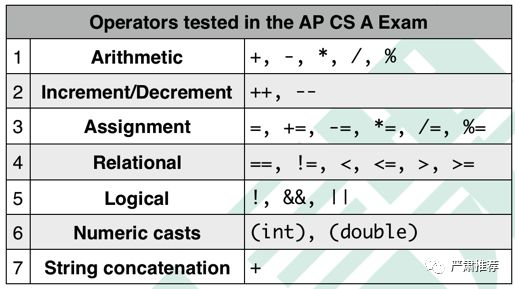1. Arithmetic Operators

2. Increment/Decrement Operators

int a = 0;

a++;

System.out.println(a);

int a = 0;

System.out.println(a++);

int[] arr = {1, 2, 3}

int i = 0;

System.out.println(arr[i++]);

3. Assignment Operators

“=” 是最基本、最常见的一个赋值操作符。“=”会将右边的数值赋给左边的变量。

“=”可以和前面的5种算数运算符结合，结果就会形成新的赋值操作符，即+=，-=， *=， /=，%=这5种赋值操作符，我们可以把它们叫做“增强赋值操作符”或“简洁赋值操作符”，具体功能如下表所示：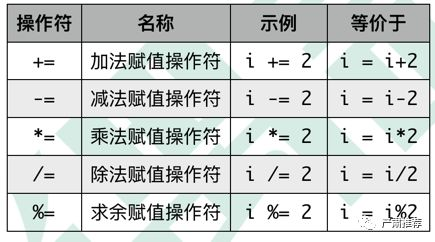i /= 2 - 3.5 * 2;

i = i / (2 - 3.5 * 2);

4. Relational Operators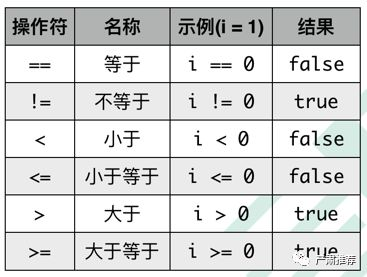5. Logical Operators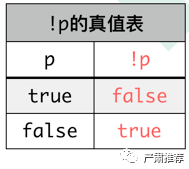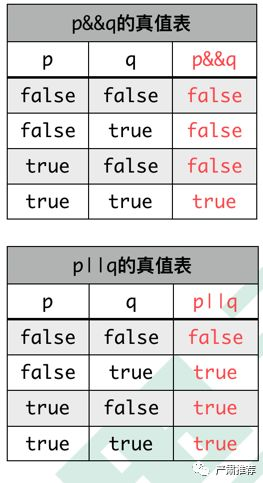boolean p = true;

boolean q = false;

if (p || (q = true))

System.out.println(q);

boolean p = true;

boolean q = false;

if (!(q && (p = false)))

System.out.println(p);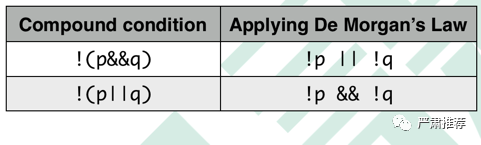6. Numeric Casts

System.out.println((int)1.9);

System.out.println((double)1/4)；

7. String Concatenation

String str = “Hello”;

System.out.println(str + “ ” + “SAT小帮手!”);

System.out.println(“Java”+2018);

9 + 2*5 > 3 * (5 - 2) + 1 && (11 - 2 > 7)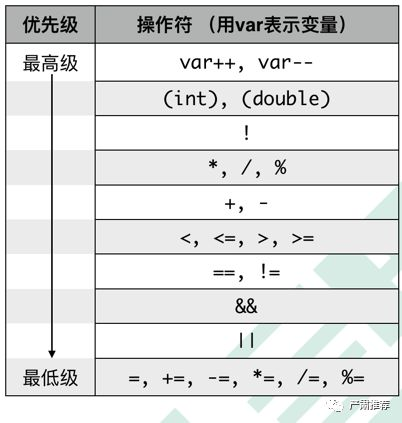i + j - k 等价于 (i + j) - k。

System.out.println(2018 + 1 + “SAT”);

System.out.println(“SAT” + 2018 +1);

int a=1; int b= 2;

a = b = 3;

System.out.println(a);

System.out.println(b);

3

3

int a = 4; int b = 5;

a %= b %= 3;

System.out.println(a);

System.out.println(b);

---------

09:00-11:00